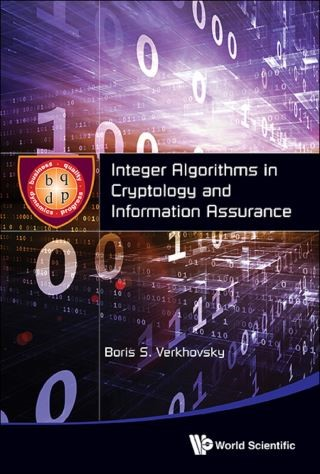## Integer Algorithms In Cryptology And Information Assurance• Description
• Author
• Info
• Reviews

### Description

Integer Algorithms in Cryptology and Information Assurance is a collection of the author's own innovative approaches in algorithms and protocols for secret and reliable communication. It concentrates on the “what” and “how” behind implementing the proposed cryptographic algorithms rather than on formal proofs of “why” these algorithms work.

The book consists of five parts (in 28 chapters) and describes the author's research results in:

• Innovative methods in cryptography (secret communication between initiated parties);
• Cryptanalysis (how to break the encryption algorithms based on computational complexity of integer factorization and discrete logarithm problems);
• How to provide a reliable transmission of information via unreliable communication channels and;
• How to exploit a synergetic effect that stems from combining the cryptographic and information assurance protocols.

This text contains innovative cryptographic algorithms; computationally efficient algorithms for information assurance; new methods to solve the classical problem of integer factorization, which plays a key role in cryptanalysis; and numerous illustrative examples and tables that facilitate the understanding of the proposed algorithms.

The fundamental ideas contained within are not based on temporary advances in technology, which might become obsolete in several years. The problems addressed in the book have their own intrinsic computational complexities, and the ideas and methods described in the book will remain important for years to come.

Contents:
• Introductory Notes on Security and Reliability
• Enhanced Algorithm for Modular Multiplicative Inverse
• Multiplication of Large-Integers Based on Homogeneous Polynomials
• Deterministic Algorithms for Primitive Roots and Cyclic Groups with Mutual Generators
• Primality Testing via Complex Integers and Pythagorean Triplets
• Algorithm Generating Random Permutation
• Extractability of Square Roots and Divisibility Tests
• Extraction of Roots of Higher Order in Modular Arithmetic
• Public-Key Cryptography Based on Square Roots and Complex Modulus
• Cubic Roots of Complex Integers and Encryption with Digital Isotopes
• Exponentiation-Free Accelerated Encryption/Decryption Protocol
• Cryptocol Based on Three-Dimensional Elliptic Surface
• Multi-Parametric Cryptography for Rapid Transmission of Information
• Scheme for Digital Signature that Always Works
• Hybrid Cryptographic Protocols Providing Digital Signature
• Control Protocols Providing Information Assurance
• Information Assurance Based on Cubic Roots of Integers
• Simultaneous Information Assurance and Encryption Based on Quintic Roots
• Modular Equations and Integer Factorization
• Counting Points on Hyper-Elliptic Curves and Integer Factorization
• Integer Factorization via Constrained Discrete Logarithm Problem
• Decomposability of Discrete Logarithm Problems
• Detecting Intervals and Order of Point on Elliptic Curve
• Generalization of Gauss Theorem and Computation of Complex Primes
• Space Complexity of Algorithm for Modular Multiplicative Inverse
• New Algorithm Can Be Computed
• Search for Period of Odd Function
• Optimized Search for Maximum of Function on Large Intervals
• Topological Design of Satellite Communication Networks

Readership: Integer Algorithms in Cryptology and Information Assurance would be a book of interest for researchers and developers working on telecommunication security and/or reliability, in industries such as business and banking, national security agencies, militaries, interplanetary space exploration, and telemedicine. Faculty members in Computer Science, Electrical Engineering, Information Technology, Bio-Engineering and Applied Mathematics Departments, who are planning to teach advanced courses in cryptography; graduate and PhD students; advanced undergraduate students of the same fields; and various national cryptographic societies would also find this book useful.
Key Features:
• Innovative cryptographic algorithms
• Computationally efficient algorithms for information assurance
• New methods to solve the classical problem of integer factorization, which plays a key role in cryptanalysis
• Numerous illustrative examples and tables that facilitate understanding of the proposed algorithms
• The style of the book, which concentrates on constructive description of what and how to implement the proposed cryptographic algorithms rather than on formal proofs of why these algorithms work